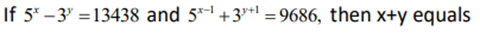# If 5^x – 3^y = 13438 - Quantitative Aptitude - Equation

## CAT 2019 - Slot 2 - Quantitative Aptitude - Equation - Question 2 - If 5^x – 3^y = 13438

Q. 2 :Given,

5^x – 3^y = 13438—–(1)

5^(x-1) + 3^(y+1) = 9686—-(2)

From equation 2, we get

5^x /5 + 3. 3^y = 9686

Multiplying equation 1 by 3 and adding, we get

5^x = 15625

So, x = 6

Putting x in equation 1, we get

3^y = 2187

Y = 7

So, x+y = 13

### Past Year Question Paper & SolutionsCounselling Session
By IIM Mentor

#### Free Material Area